×#### Thank you for registering.

One of our academic counsellors will contact you within 1 working day.

Click to Chat

1800-1023-196

+91-120-4616500

CART 0

• 0

MY CART (5)

Use Coupon: CART20 and get 20% off on all online Study Material

ITEM
DETAILS
MRP
DISCOUNT
FINAL PRICE
Total Price: Rs.

There are no items in this cart.
Continue Shopping• Complete JEE Main/Advanced Course and Test Series
• OFFERED PRICE: Rs. 15,900
• View Details

```Practical Geometry Exercise 18.1

Question: 1

Construct a quadrilateral ABCD in which AB = 4.4 cm, BC = 4 cm, CD = 6.4 cm, DA = 3.8 cm and BD = 6.6 cm.

Solution:First, we draw a rough sketch of the quadrilateral ABCD and write down its dimensions along the sides.

We may divide the quadrilateral into two constructible triangles ABD and BCD.

Steps of Construction:

Step I: Draw BD = 6.6 cm

Step II: With B as the center and radius BC = 4 cm, draw an arc.

Step III: With D as the center and radius 6.4 cm, draw an arc to intersect the arc drawn in Step II at C.

Step IV: With B as the center and radius 4.4 cm, draw an arc on the side BD opposite to that of C.

Step V: With D as the center and radius 3.8 cm, draw an arc to intersect the arc drawn in Step IV at A.

Step VI: Join BA, DA, BC and CD The quadrilateral ABCD so obtained is the required quadrilateral.

Question: 2

Construct a quadrilateral ABCD such that AB = BC = 5.5 cm, CD = 4 cm, DA = 6.3 cm and AC = 9.4 cm. Measure BD.

Solution: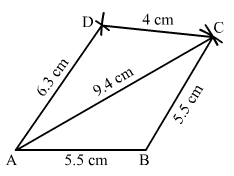Steps of Construction:

Step I: Draw AB = 5.5 cm

Step II: With B as the center and radius BC = 5.5 cm, draw an arc.

Step III: With A as the center and radius AC = 9.4 cm, draw an arc to intersect the arc drawn in Step II at C.

Step IV: With C as the center and radius CD = 4 cm, draw an arc.

Step V: With A as the center and radius AD = 6.3 cm, draw an arc to intersect the arc drawn in Step IV at D.

Step VI: Join DA, BC, AC, and CD.

Question: 3

Construct a quadrilateral XYZW in which XY = 5 cm, YZ = 6 cm, ZW = 7 cm, WX = 3 cm and XZ = 9 cm.

Solution: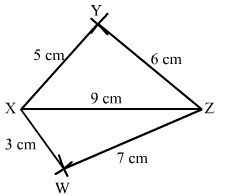Steps of Construction:

Step I: Draw XZ = 9 cm

Step II: With X as the center and radius 5 cm, draw an arc above XZ.

Step III: With Z as the center and radius 6 cm, draw an arc to intersect the arc drawn in Step II at Y above XZ.

Step IV: With Z as the center and radius 7 cm, draw an arc below XZ.

Step V: With X as the center and radius 3 cm, draw an arc to intersect the arc drawn in Step IV at W below XZ.

Step VI: Join XY, YZ, ZW, and XW.

Question: 4

Construct a parallelogram PQRS such that PQ = 5.2 cm, PR = 6.8 cm and QS = 8.2 cm.

Solution: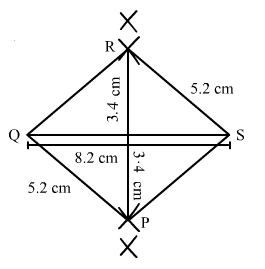In a parallelogram opposite sides are equal.

Thus, we have to construct a quadrilateral PQRS in which PQ = 5.2 cm, PR = 6.8 cm and QS = 8.2 cm.

Steps of Construction:

Step I: Draw QS = 8.2 cm

Step II: With Q as the center and radius 5.2 cm, draw an arc.

Step III: With S as the center and radius 5.2 cm, draw an arc to intersect the arc drawn in Step II at C.

Step IV: With P as the center and radius 6.8 cm.

Step V: With Q as the center and radius 5.2 cm, draw an arc to intersect the arc drawn in Step IV at A.

Step VI: Join QR, QP, PS, and SR.

Question: 5

Construct a rhombus with side 6 cm and one diagonal 8 cm. Measure the other diagonal.

Solution: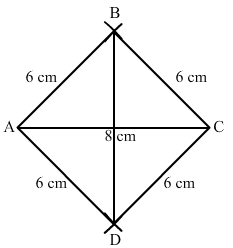Steps of Construction:

Step 1: Draw AC = 8 cm.

Step 2: With A as the centre and radius = 6 cm, draw arcs on both sides.

Step 3: With C as the center and radius = 6 cm, draw arcs on both sides, intersecting the previous arcs at points B and D.

Step 4: Join BD = 8.9cm.

Thus, ABCD is the required rhombus.

Question: 6

Construct a kite ABCD in which AB = 4 cm, BC = 4.9 cm and AC = 7.2 cm.

Solution:Steps of Construction:

Step I: Draw AC = 7.2 cm.

Step II: With A as the centre and radius 4cm, draw arcs on both sides of the line segment AC.

Step III: With C as the centre and radius 4.9 cm, draw arcs on both sides of AC intersecting the previous arcs of step II at B and D.

Step IV: Join BA, DA, BC and CD.

Thus, the quadrilateral ABCD so obtained is the required kite.

Question: 7

Construct, if possible, a quadrilateral ABCD given AB = 6 cm, BC = 3.7 cm, CD = 5.7 cm, AD = 5.5 cm and BD = 6.1 cm. Give reasons for not being able to construct it, if you cannot.

Solution: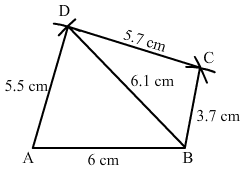Steps of Construction:

Step I: Draw AB = 6 cm.

Step II: With A as the center and radius 5.5 cm, draw an arc.

Step III: With B as the center and radius 6.1 cm, draw an arc to intersect the arc drawn in Step II at D.

Step IV: With B as the centre and radius 3.7 cm, draw an arc on the side.

Step V: With D as the centre and radius 5.7 cm, draw an arc to intersect the arc drawn in Step IV at C.

Step VI: Join BD, DA, BC and CD.

Question: 8

Construct, if possible, a quadrilateral ABCD in which AB = 6 cm, BC = 7 cm, CD = 3 cm, AD = 5.5 cm and AC = 11 cm. Give reasons for not being able to construct, if you cannot. (Not possible, because in triangle ACD, AD + CD <AC).

Solution:

Such a quadrilateral cannot be constructed because, in a triangle, the sum of the length of its two sides must be greater than that of the third side

But here in triangle ACD,

AD + CD = 5.5 + 3 = 8.5 cm

and AC = 11 cm

i.e., AD + CD < AC, which is not possible.

So, the construction is not possible.
```### Course Features

• 728 Video Lectures
• Revision Notes
• Previous Year Papers
• Mind Map
• Study Planner
• NCERT Solutions
• Discussion Forum
• Test paper with Video Solution